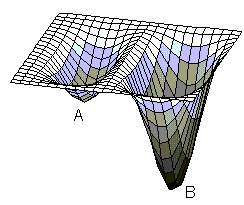Geometry Optimization Geometry Optimization is a systematic method of rearranging atoms such that the net forces on the structure of the molecule equal zero. This is best understood in terms of the values of potential energy surfaces. If given a 3 dimensional space where one dimension, z, represents the value of the potential energy surface and the other two dimensions represent two parameters, such as x and y coordinate positions of a specific atom then, the 3 dimensional space could look something like:Both points A and B represent optimized geometries. This means that optimized geometries represent local minimums of the potential energy surface. However, the most likely configuration of a molecule in nature is the global minimum, point B. Thus, a geometry optimization usually generates a stable structure but not the most stable structure for a molecule.